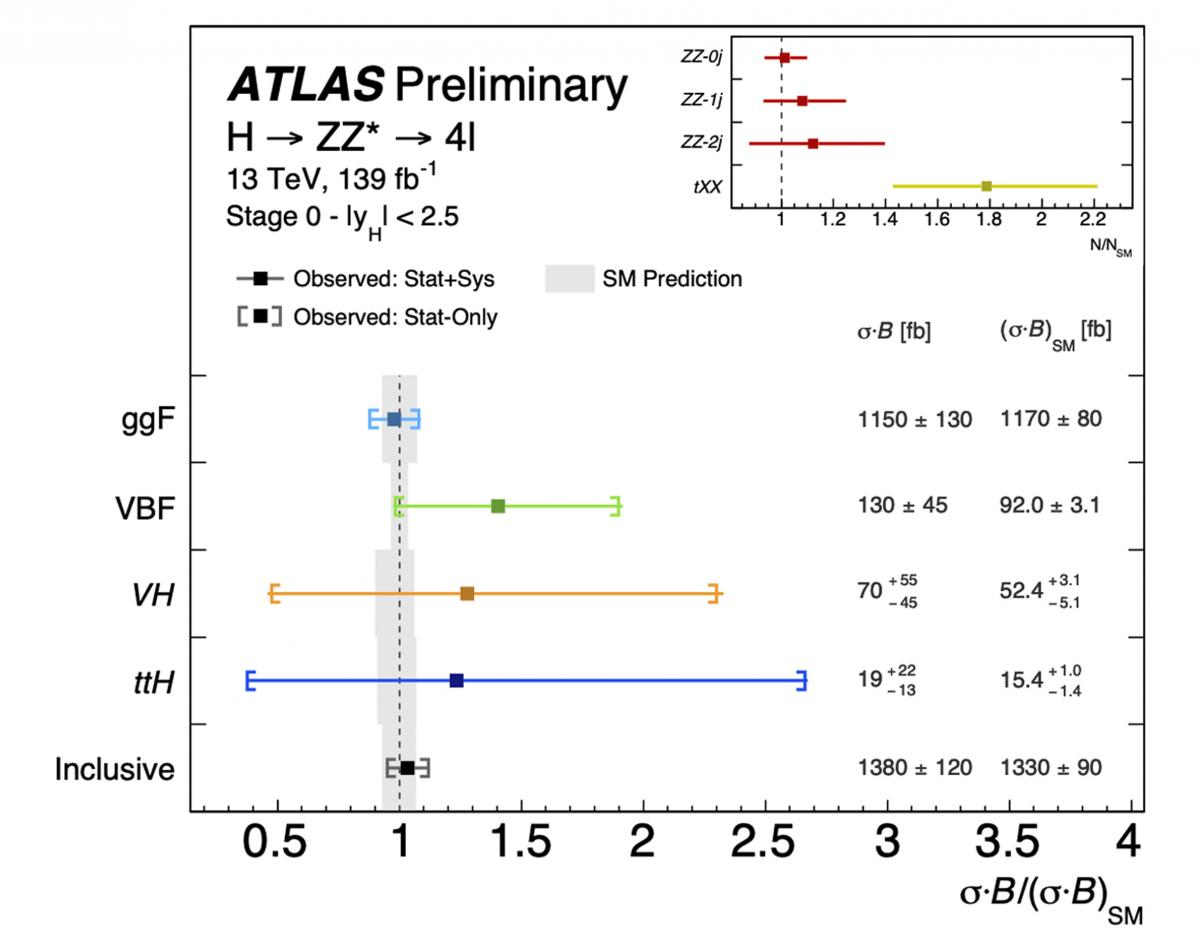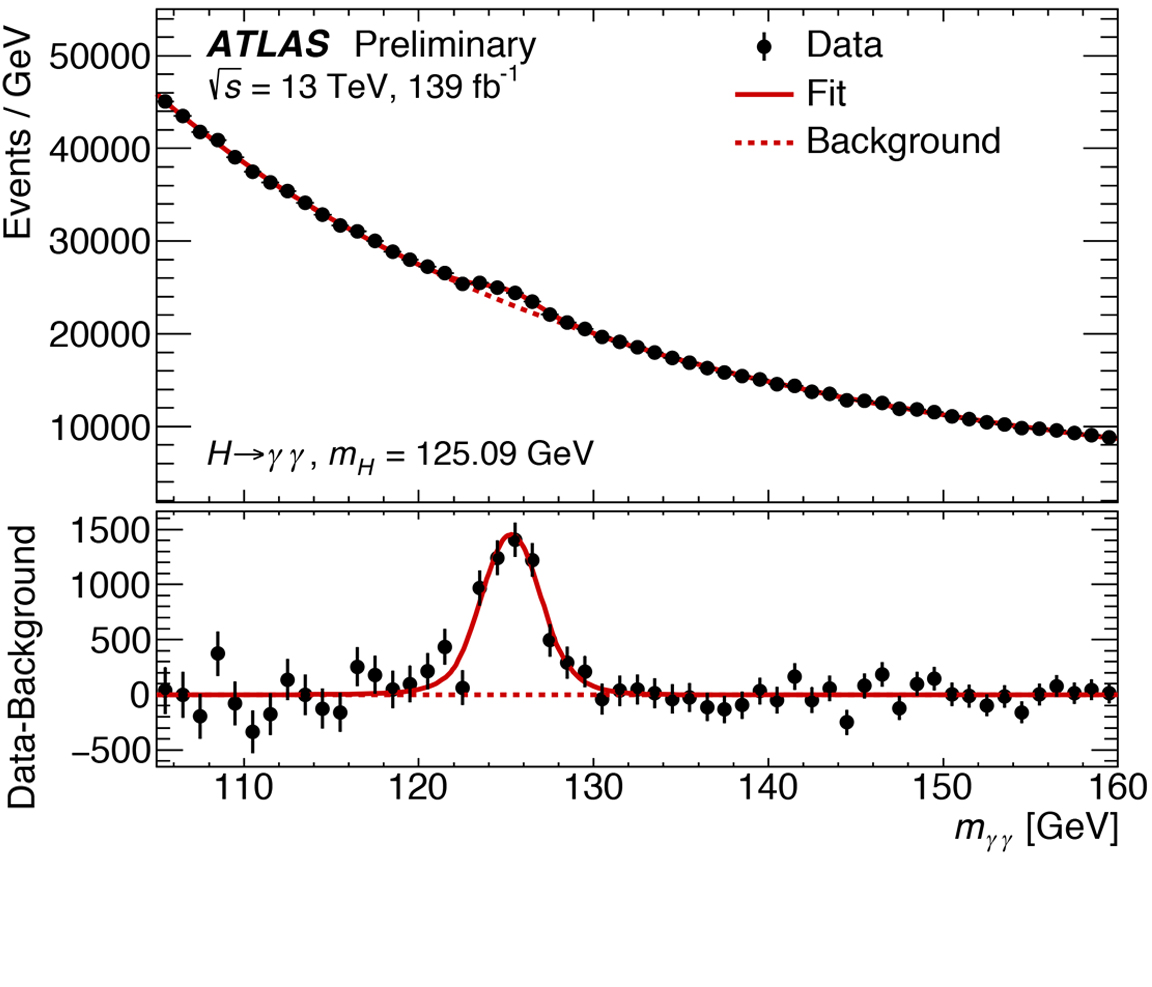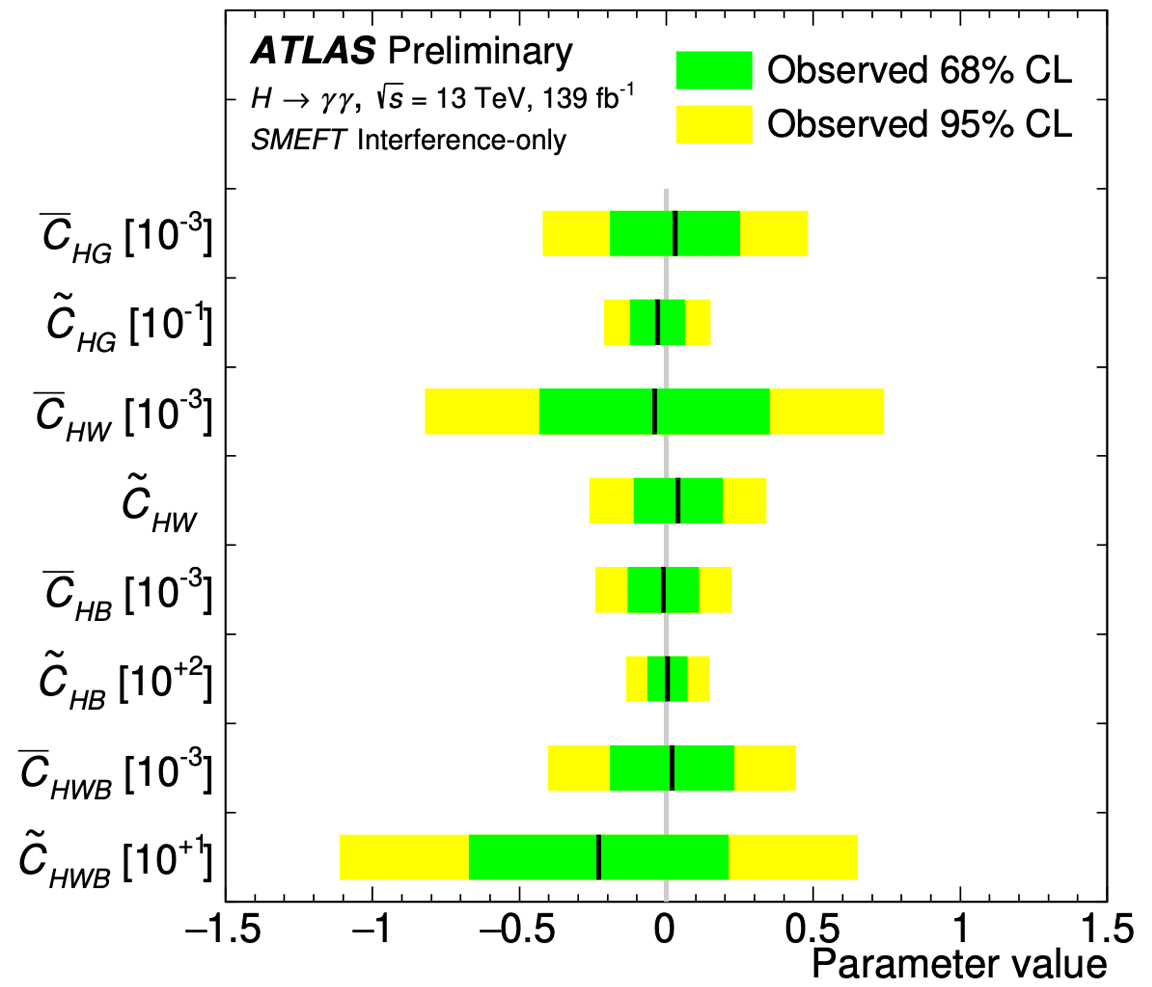Event display of a two-electron two-muon ZH candidate. The Higgs candidate can be seen on the left with the two leading electrons represented by green tracks and green EM calorimeter deposits (pT = 22 and 120 GeV), and two subleading muons indicated by two red tracks (pT = 34 and 43 GeV). Recoiling against the four lepton candidate in the left hemisphere is a dimuon pair in the right hemisphere indicated by two red tracks (pT = 139 and 42 GeV) and an invariant mass of 91.5 GeV, which agrees well with the mass of the Z boson. (Image: ATLAS Collaboration/CERN)

This week, at the European Physical Society Conference on High-Energy Physics (EPS-HEP) in Ghent, Belgium, the ATLAS Collaboration at CERN released new measurements of Higgs boson properties using the full LHC Run 2 dataset. Critically, the new results examine two of the Higgs boson decays that led to the particle’s discovery in 2012: H→ZZ*→4ℓ, where the Higgs boson decays into two Z bosons, in turn decaying into four leptons (electrons or muons); and H→γγ where the Higgs boson decays directly into two photons.

Although rare, these channels are easily identified and well measured in the ATLAS detector, allowing physicists to study properties of the Higgs boson with great precision. In particular, they provide new measurements of the Higgs boson’s transverse momentum, which can be used to probe different Higgs production mechanisms and possible deviations from the Standard Model interactions.

### Going for gold: new insight into the four-lepton channel

The H→ZZ*→4ℓ decay is the so-called “golden channel” of the Higgs boson, as it has the clearest and cleanest signature of all the possible Higgs boson decay modes. Thanks to the increased luminosity of Run 2, ATLAS recorded around 300 “golden channel” candidate events between 2015 and 2018, of which one third are expected to be due to ZZ background processes. The invariant mass spectrum for selected four-lepton events for the full Run 2 data can be seen in Figure 1.

In addition to the increased number of events collected, ATLAS physicists made improvements to their analysis. While the ZZ background rate was previously estimated with simulations, associated with a theoretical uncertainty, the new ATLAS result uses data to directly assess the background contribution. While keeping the total uncertainty on the background about the same, this has significantly reduced the theoretical uncertainty and model dependence of the measurement.Figure 1: Distribution of the invariant mass of the four leptons selected in the ATLAS measurement of H→ZZ*→4ℓ using the full Run 2 dataset. The Higgs boson corresponds to the excess of events (blue) over the non-resonant ZZ* background (red) at 125 GeV. A rare Z boson decay into four leptons can also be observed at about 91 GeV. (Image: ATLAS Collaboration/CERN)Figure 2: The Higgs production cross sections times the Higgs branching fraction into a pair of Z bosons, σ•B, as measured in the four-lepton decay channel. These are normalised by the Standard Model expected values. The vertical line at one is the Standard Model expectation with the grey band corresponding to the theory uncertainties on this expectation. The measured data points agree well within their uncertainties with the Standard Model. (Image: ATLAS Collaboration/CERN)

The ATLAS team also introduced Deep Learning Neural Networks to distinguish which of the Higgs boson events came from which production mechanisms. This technique allows the ATLAS team to improve their identification of whether a Higgs boson had been produced by the common fusion of a pair of gluons (ggF – accounting for 87% of Higgs boson decays), or from the rarer fusion of two W or Z vector bosons (VBF – 7% of decays) or the radiation from a W or Z boson (VH – 4%). Once successfully identified, the ATLAS physicists could then measure the production cross section for each.

The VBF and VH production modes can be fairly well distinguished by the separation and mass of “jets” of particles they produce. For VBF, the vector bosons are radiated by two quarks, which form energetic jets in the detector along the beam direction and in opposite hemispheres. Meanwhile, the VH production mode also produces two jets, with either the mass of the W (80 GeV) or Z (91 GeV) boson.

However, the gluons in the ggF production can also radiate extra jets, thus imitating VBF and VH jet pairs. This is where Deep Learning Neural Networks come in. They have proven to be flexible enough to simultaneously separate ggF, VBF and VH with less overlap than previous machine learning techniques. The Higgs production cross sections as measured in the four-lepton channel can be seen in Figure 2, where there is a 20% improvement on the VBF cross-section measurement thanks to the Neural Network technique.

### Two lights to see the Higgs: studying the two-photon channel

ATLAS physicists also incorporated new and improved analysis techniques in their study of the Higgs boson decay to a pair of photons (H→γγ). In particular, the enhanced photon identification and jet energy calibration led to reduced associated systematic uncertainties. The electromagnetic shower shape criteria used to identify photons and suppress unwanted photon candidates from hadron decays have now been optimised in sub-ranges of the photon transverse momentum, as the showers generated in the detector depends on the photon energy. This led to improvements in the sensitivity of a few percent.Figure 3: Distribution of the invariant mass of the two photons elected in the ATLAS measurement of H→γγ using the full Run 2 dataset. The Higgs boson corresponds to the excess of events observed at 125 GeV with respect to the non-resonant background (dashed line). (Image: ATLAS Collaboration/CERN)Figure 4: A combined fit to five of the measured H→γγ differential cross-sections is used to constrain the coupling coefficients of non-standard interactions between the Higgs boson and the gauge bosons, in the context of an effective Lagrangian. Limits are derived assuming a single coefficient is different from zero. (Image: ATLAS Collaboration/CERN)

Physicists measured several differential cross sections for observables sensitive to Higgs boson production and decay, including kinematic distributions of the jets produced in association with the Higgs boson. Beyond-the-Standard-Model interactions between the Higgs boson and the gauge bosons are expected to modify these variables, providing an excellent test for new physics. The inclusive and differential cross-section measurements, determined from the yields of events in the signal peak in the diphoton invariant mass distribution (see Figure 3) were found to be in good agreement with Standard Model predictions. ATLAS physicists used these measurements to constrain the strength of hypothetical beyond-the-Standard Model interactions of the Higgs boson with the gauge bosons (see Figure 4).Figure 5: Differential cross section for the transverse momentum (pT,H) of the Higgs boson from the two individual channels (H→ZZ*→4ℓ, H→γγ) and their combination. (Image: ATLAS Collaboration/CERN)

Moreover, ATLAS physicists were able to examine the interaction between the Higgs boson and the charm quark. The Higgs boson has not yet been seen decaying to charm quarks, which is predicted in the Standard Model to have twenty times lower rate than decays to bottom quarks, observed for the first time by ATLAS and CMS in 2018. However, if the strength (or “coupling”) of the Higgs interaction to the charm quark were much larger than expected due to some new physics process, this would affect the measured momentum distribution of the Higgs boson. Physicists looked for the signature of this effect: an excess of the data compared to the theory expectation in the low Higgs boson momentum region (see Figure 5). Such an excess was not observed in the data.

### Combined insight

The total Higgs boson production cross section was measured to be 56.7 ± 6.3 pb with the H→γγ decay channel, and 54.4 ± 5.6 pb with the H→ZZ*→4ℓ channel. Combining the two channels, the total cross section is 55.4 ± 4.3 pb, in agreement with the Standard Model prediction of 55.6 ± 2.5 pb. The differential cross section for the transverse momentum of the Higgs boson in both channels also agree, as seen in Figure 5, and their combination fits with the Standard Model predictions.

Thanks to the excellent performance of the LHC and of the ATLAS detector during Run 2, ATLAS studies of the Higgs boson are moving beyond discovery, into a new era of precision measurements that further our understanding of this particle. The journey has just begun!